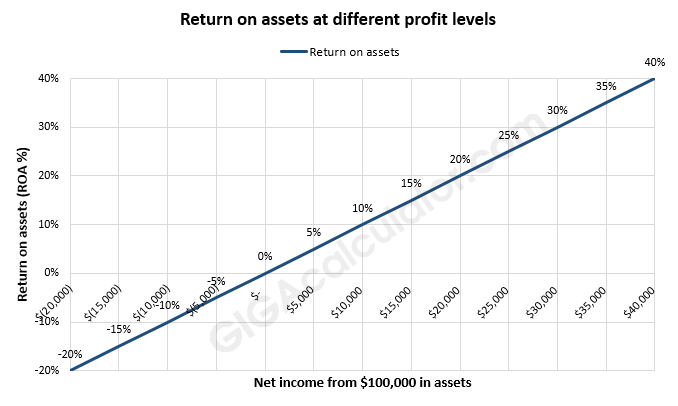# Return on Assets Calculator

Use this return on assets calculator to easily calculate ROA (return on assets) based on the net income generated and the total value of the assets of the company or project.

\$
\$
Share calculator:

Embed this tool:
get code

## ROA Formula

The formula for ROA used in our return on assets calculator is simple:

ROA = Net Income / Total Assets

Both input values are in the relevant currency while the result is a ratio. To get a percentage result simply multiply the ratio by 100.

## How to calculate return on assets?

Return on Assets (ROA) is a metric used to estimate how well a company or project makes use of its capital assets. It is calculated as the company profit relative to the total value of its assets.

Using the above formula, one needs to simply substitute the relevant values and use a calculator to arrive at the final value. For example, if the net income (profit) of a company for the fiscal year is \$100,000 and it used assets worth \$500,000 to produce it, then its return on assets is 1/5 or 20%. In other words, it returns 1 dollar of value for every 5 dollars of capital assets. As the total assets are the sum of the total liabilities and shareholder equity which can fluctuate over the year you can input their arithmetic average instead. A positive ROA means the company is increasing its assets while a negative ROA means that the company is losing capital.If you are the sole investor in the company, then the return on assets is equal to your return on investment (ROI) so you can use this return on assets calculator or a ROI calculator with to the same end.

## What is a good return on assets?

In most mature businesses with a low level of risk a 5% annual return on assets is considered pretty decent. Highly-volatile and risky investments, on the other hand, would be expected to bring in 10%, 20% or even higher return to justify the risk-adjusted cost. In the end, "good" is a subjective term that depends on the particular niche, competition, perceived risk, and investor time preference, among others.

For the above reasons ROA is a good measure for comparing companies only when they operate under relatively similar conditions: same industry, same geographical location, same legal and regulatory framework, etc. The more diverse these are, the more you need to consider other metrics on top of calculating ROA.

#### Cite this calculator & page

If you'd like to cite this online calculator resource and information as provided on the page, you can use the following citation:
Georgiev G.Z., "Return on Assets Calculator", [online] Available at: https://www.gigacalculator.com/calculators/return-on-assets-calculator.php URL [Accessed Date: 06 Jun, 2023].